# Telling the Time Quarter Past Half Past and

• Slides: 15Telling the Time: Quarter Past, Half Past and Quarter toHours and Minutes This is the hour hand. This is the minute hand. It is the shortest hand on the clock. It is longer than the hour hand.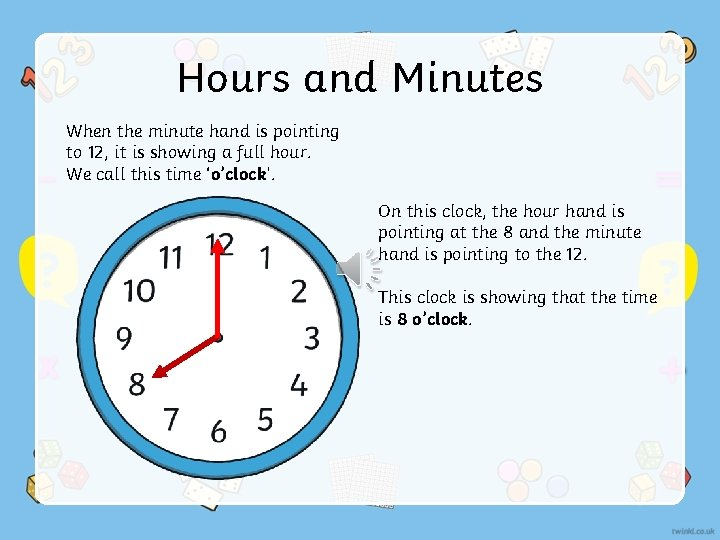Hours and Minutes When the minute hand is pointing to 12, it is showing a full hour. We call this time ‘o’clock’. On this clock, the hour hand is pointing at the 8 and the minute hand is pointing to the 12. This clock is showing that the time is 8 o’clock.What Time Is It? Which clock shows 4 o’clock?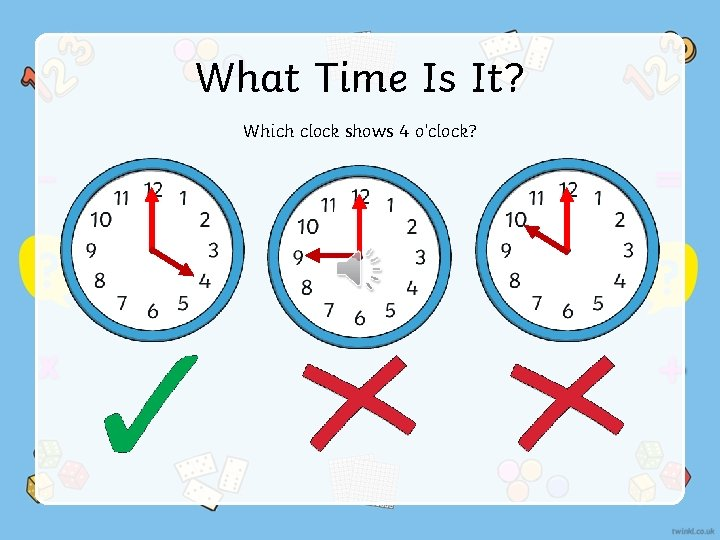What Time Is It? Which clock shows 4 o’clock?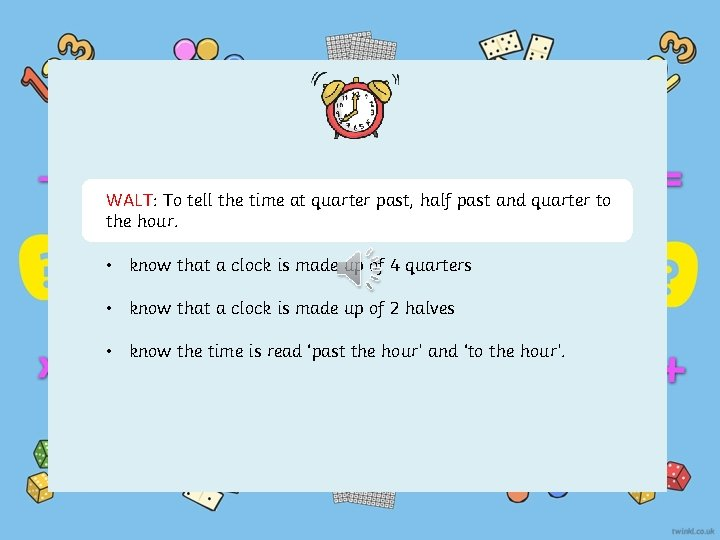WALT: To tell the time at quarter past, half past and quarter to the hour. • know that a clock is made up of 4 quarters • know that a clock is made up of 2 halves • know the time is read ‘past the hour’ and ‘to the hour’.A clock face is a full circle which is made up of 4 quarters. How many minutes make up quarter of an hour?There are 15 minutes in quarter of an hour.When we tell the time we either say it is ‘past’ or ‘to’ the hour. Quarter to Quarter past Half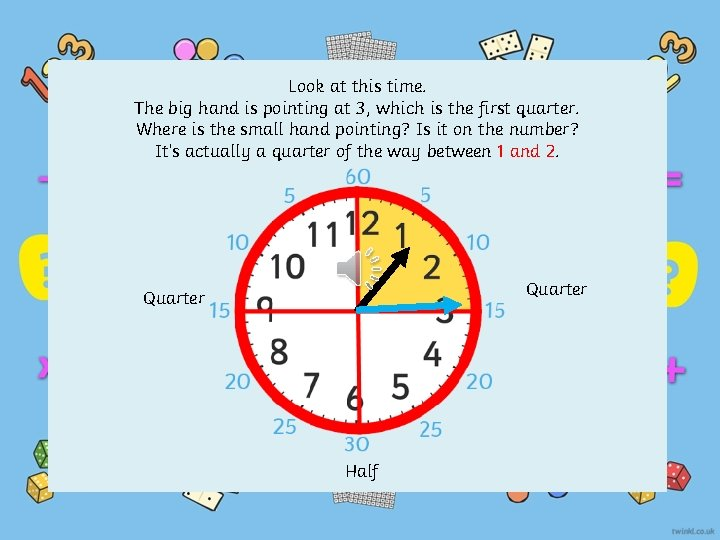Look at this time. The big hand is pointing at 3, which is the first quarter. Where is the small hand pointing? Is it on the number? It’s actually a quarter of the way between 1 and 2. Quarter HalfWhat time is this clock showing? Where is the big hand pointing? 9. Quarter to 12. Where is the little hand pointing? Three quarters of the way between 11 and 12. Quarter HalfWhat do you notice about the small hand? It is halfway between 3 and 4 What time is this clock showing? Half past three. Quarter Half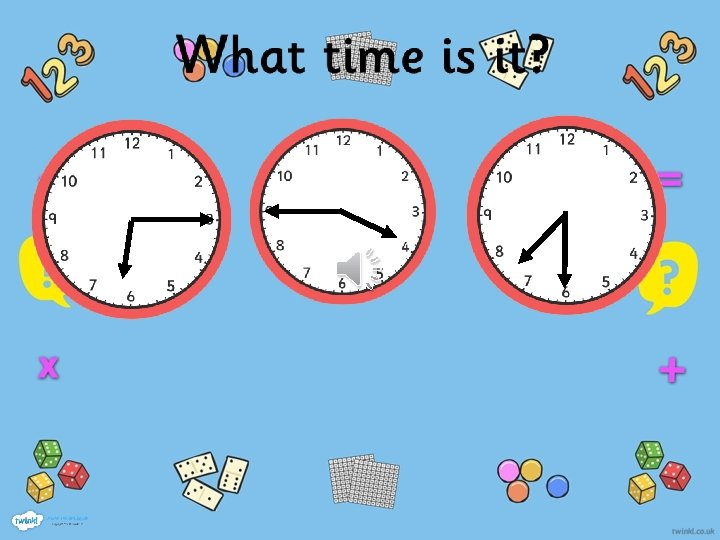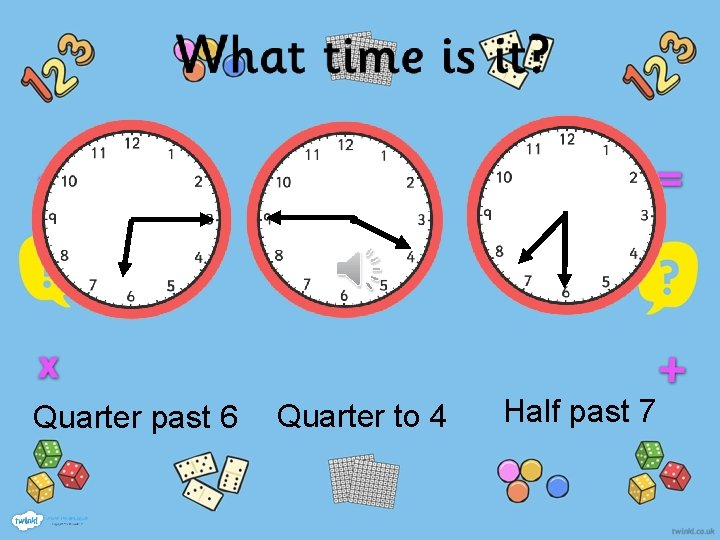Quarter past 6 Quarter to 4 Half past 7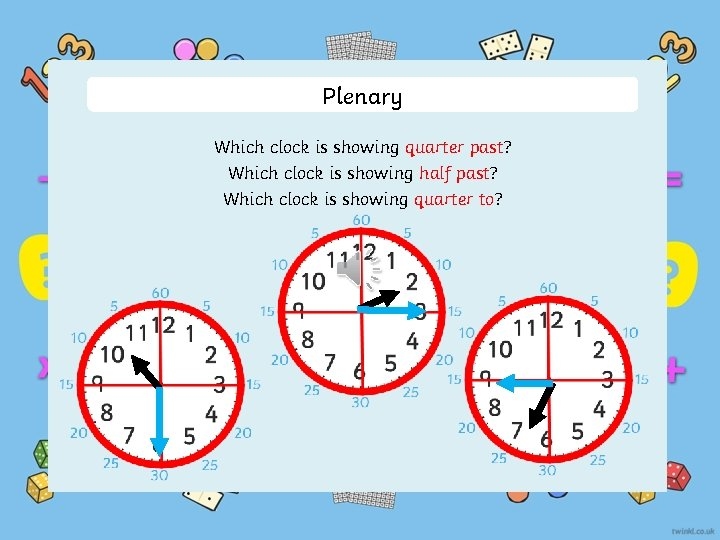Plenary Which clock is showing quarter past? Which clock is showing half past? Which clock is showing quarter to?